# ICSE Class 8 Maths Selina Solutions for Chapter 16 Understanding Shapes

Chapter 16 Understanding shapes explains about properties of quadrilaterals, angle sum property, properties of parallelograms, kinds of quadrilaterals and theorems related to them. Knowing this chapter is important because it has applications in everyday life for eg: thinking about home projects, and in various careers, like architecture. For the better understanding of the concepts related to shapes, we have provided ICSE Class 8 maths Selina Solutions Chapter 16 – Understanding Shapes. These solutions are prepared by our subject experts. The questions are solved in the comprehensive way so that students can understand them easily.

Students of Class 8 ICSE can refer to the ICSE Class 8 maths Selina Solutions for Chapter 16 Understanding Shapes from the downloadable link provided below: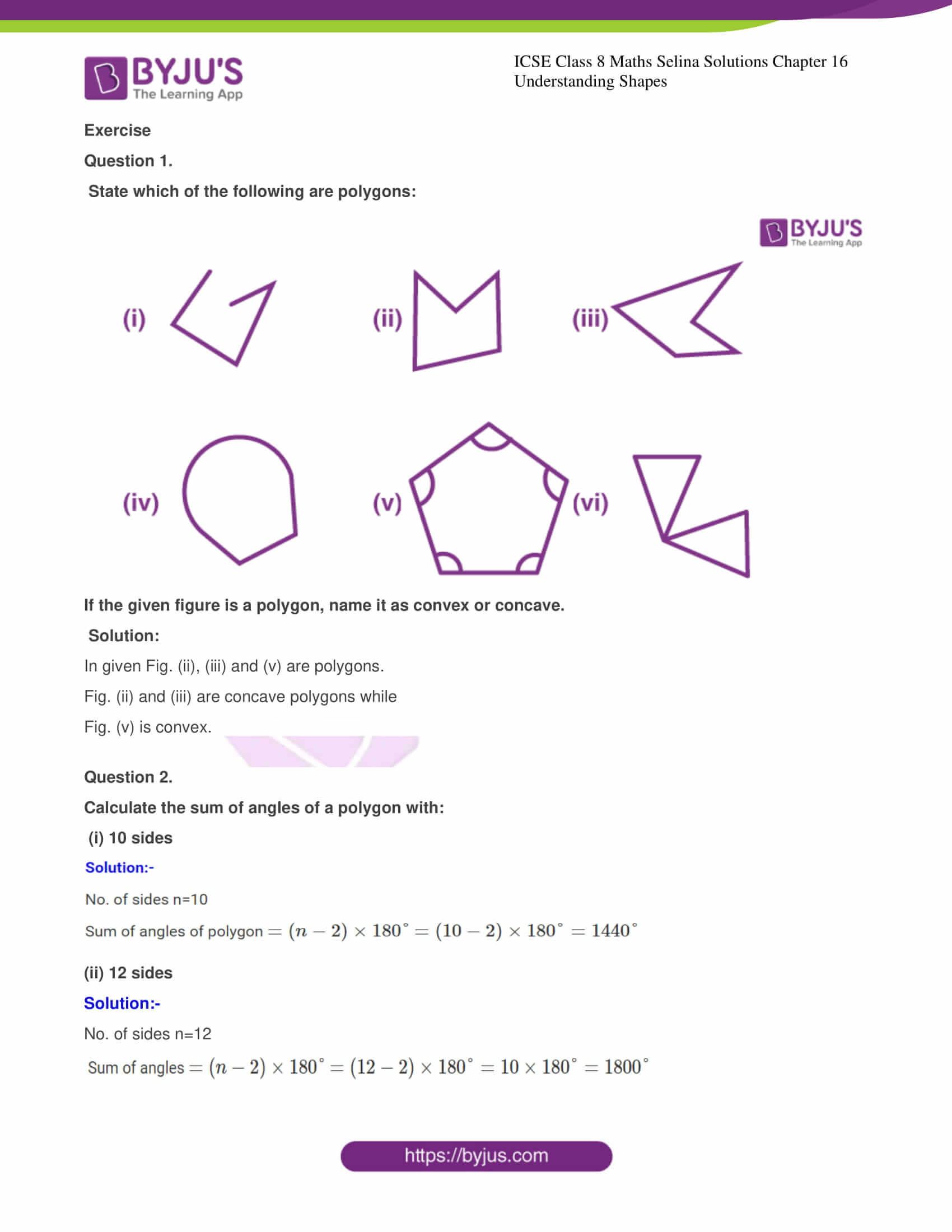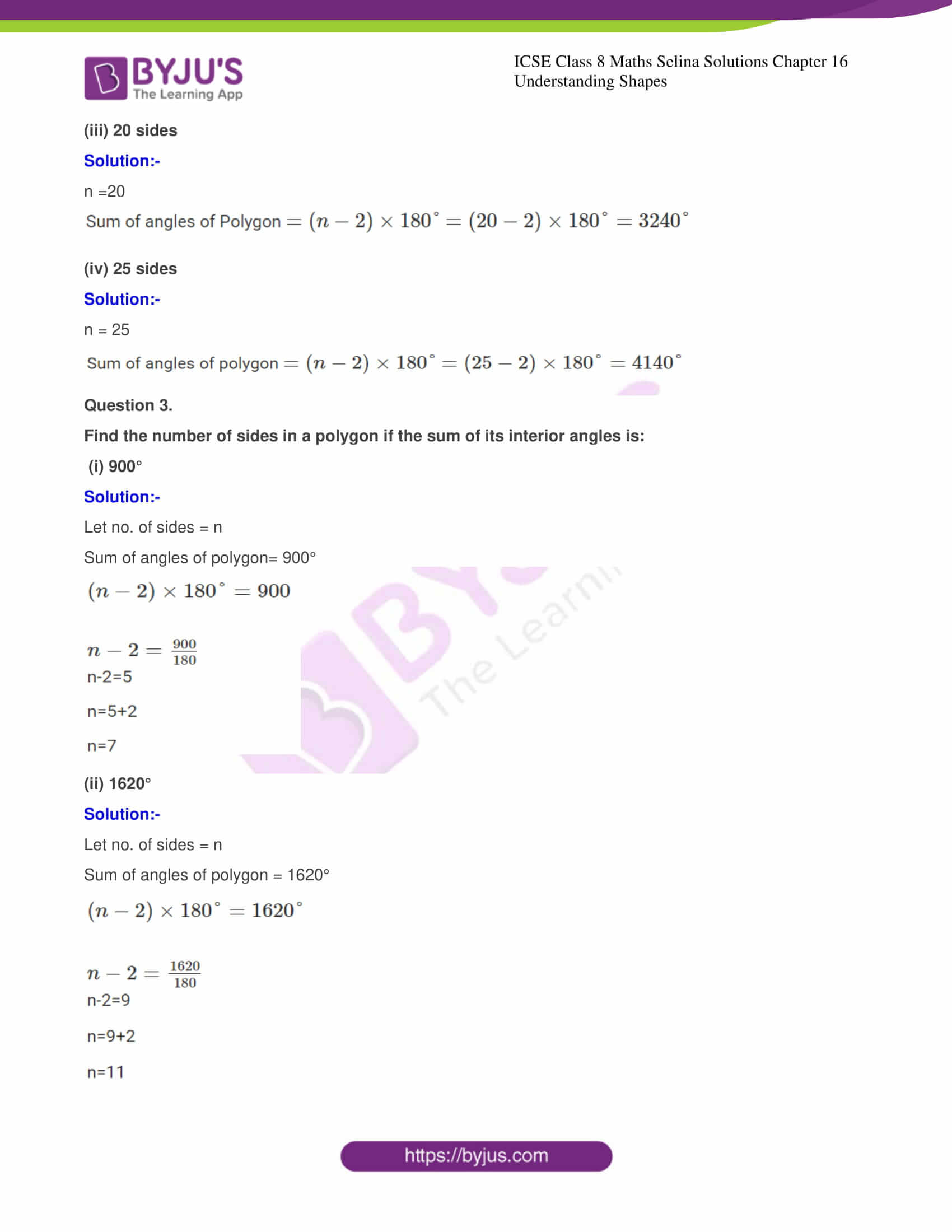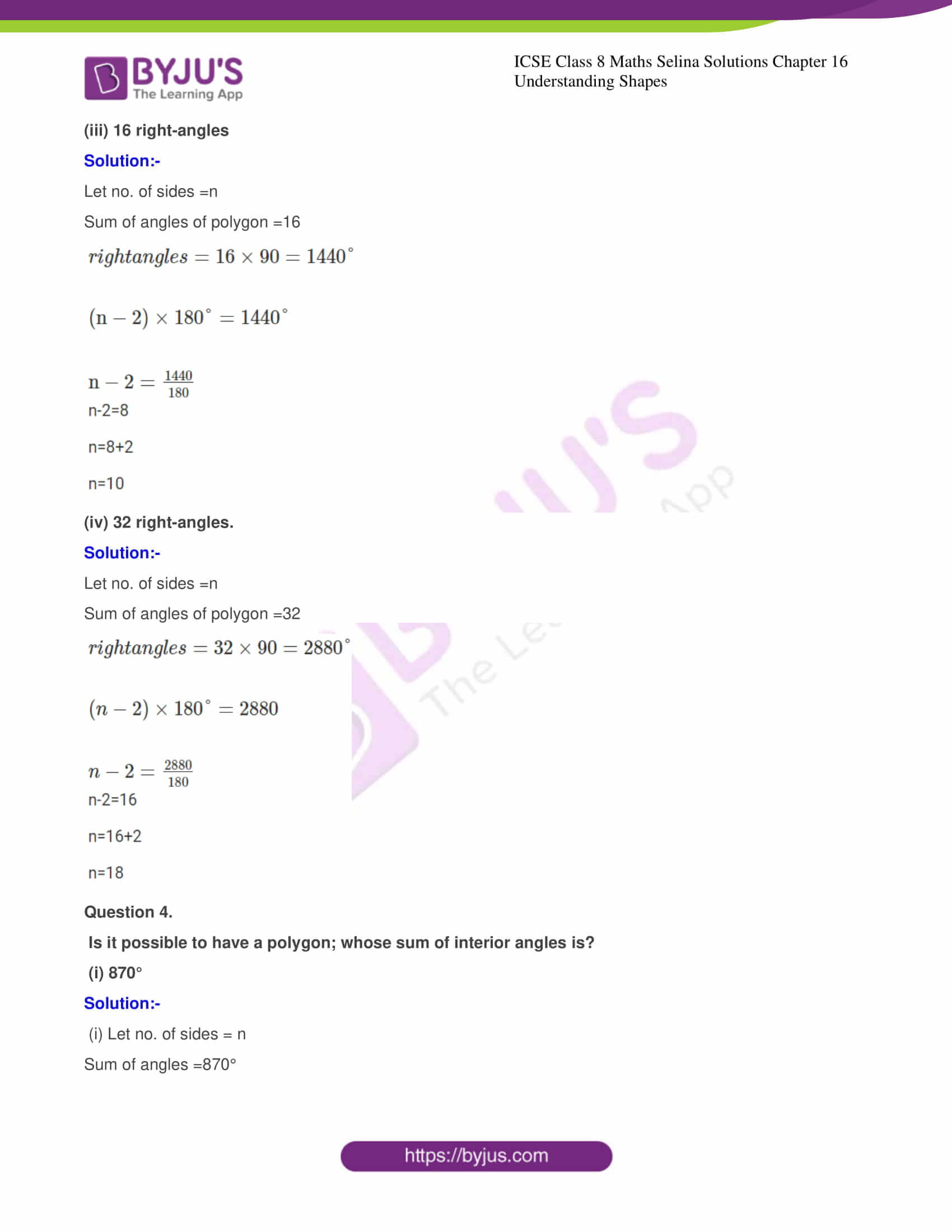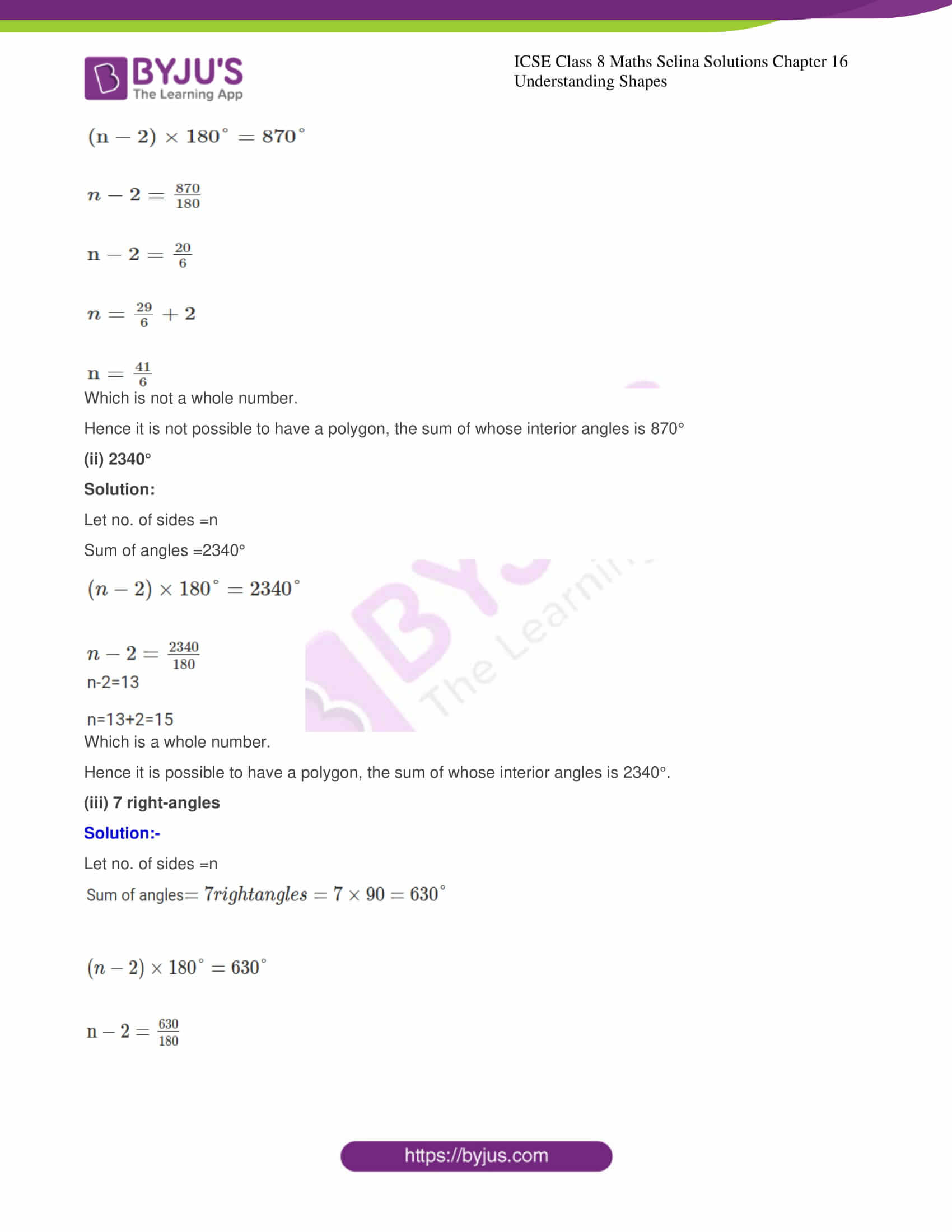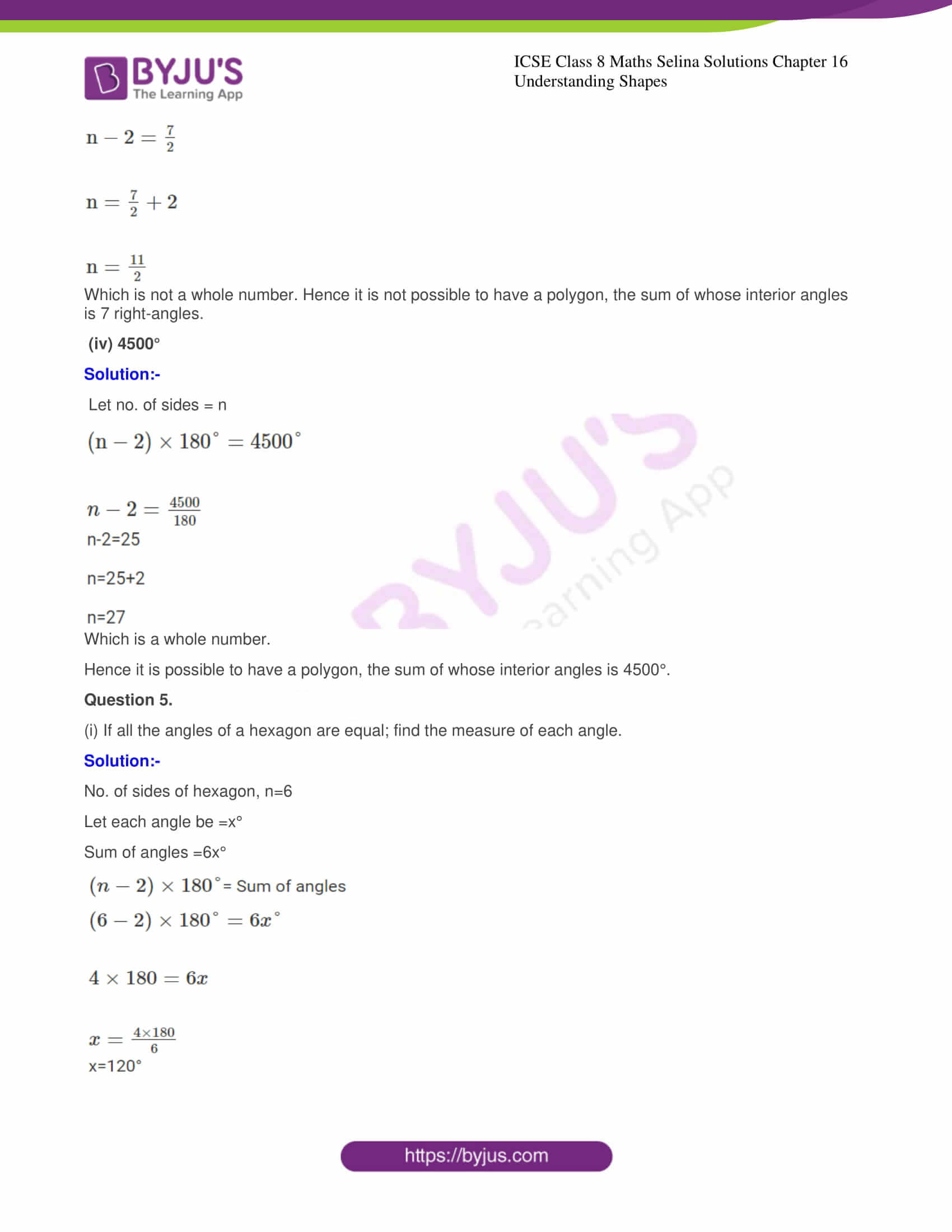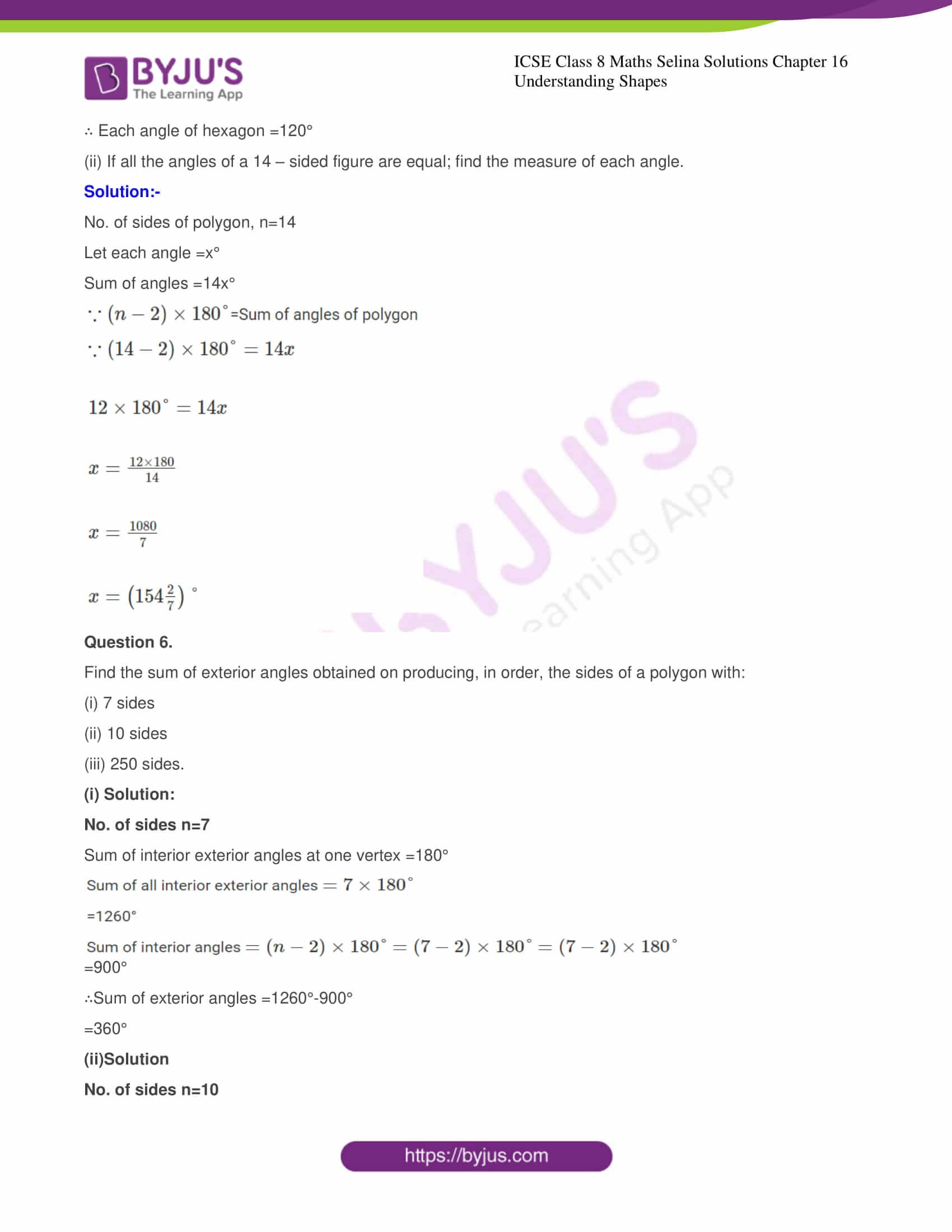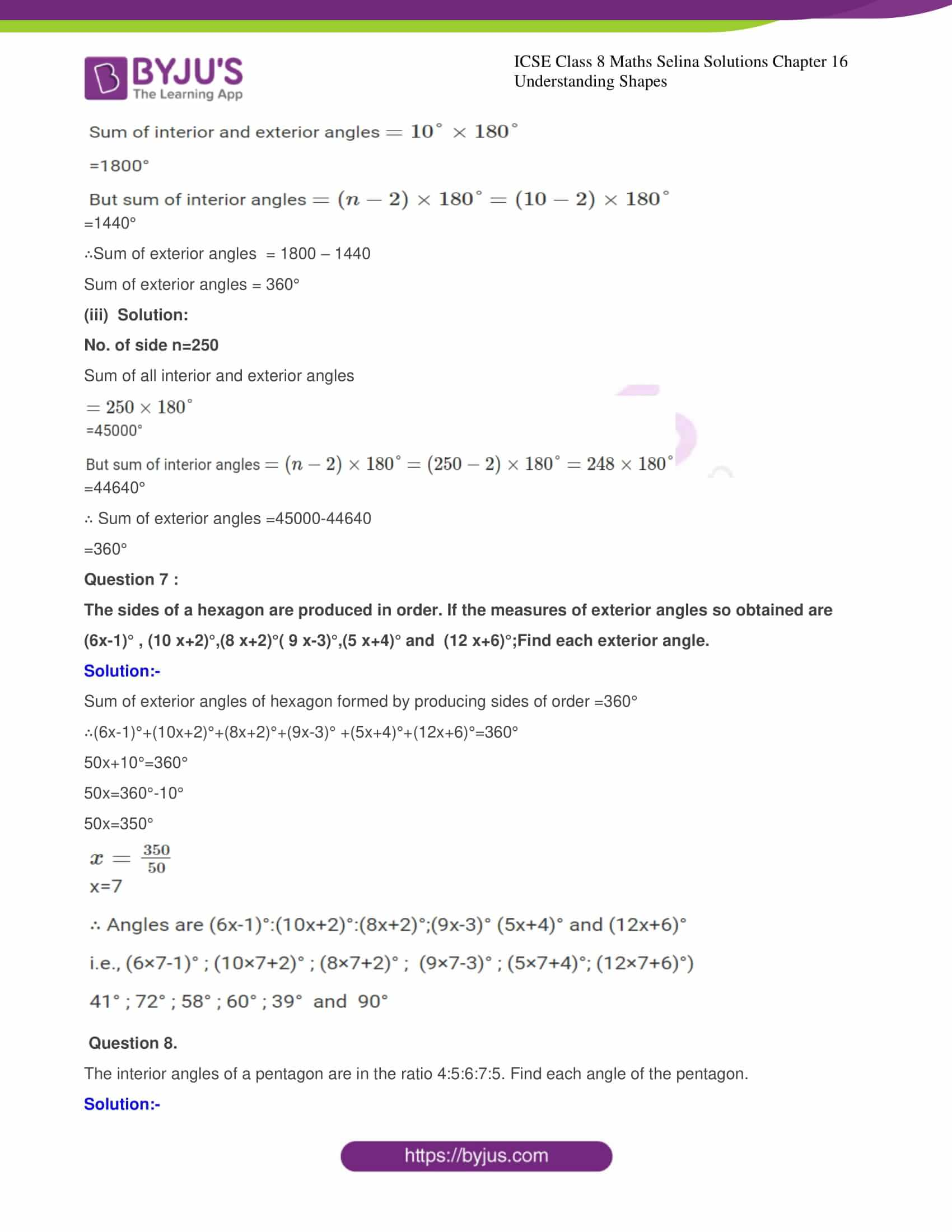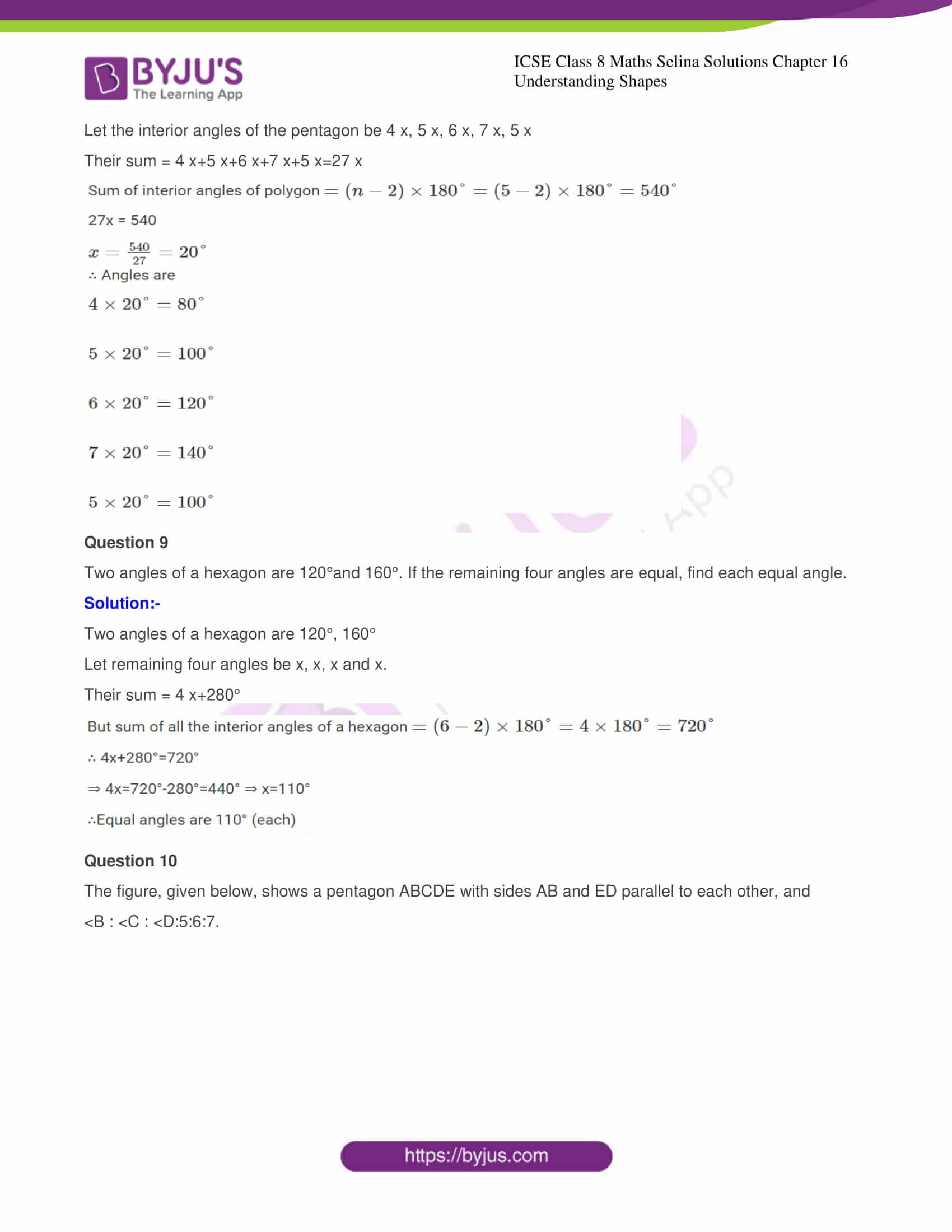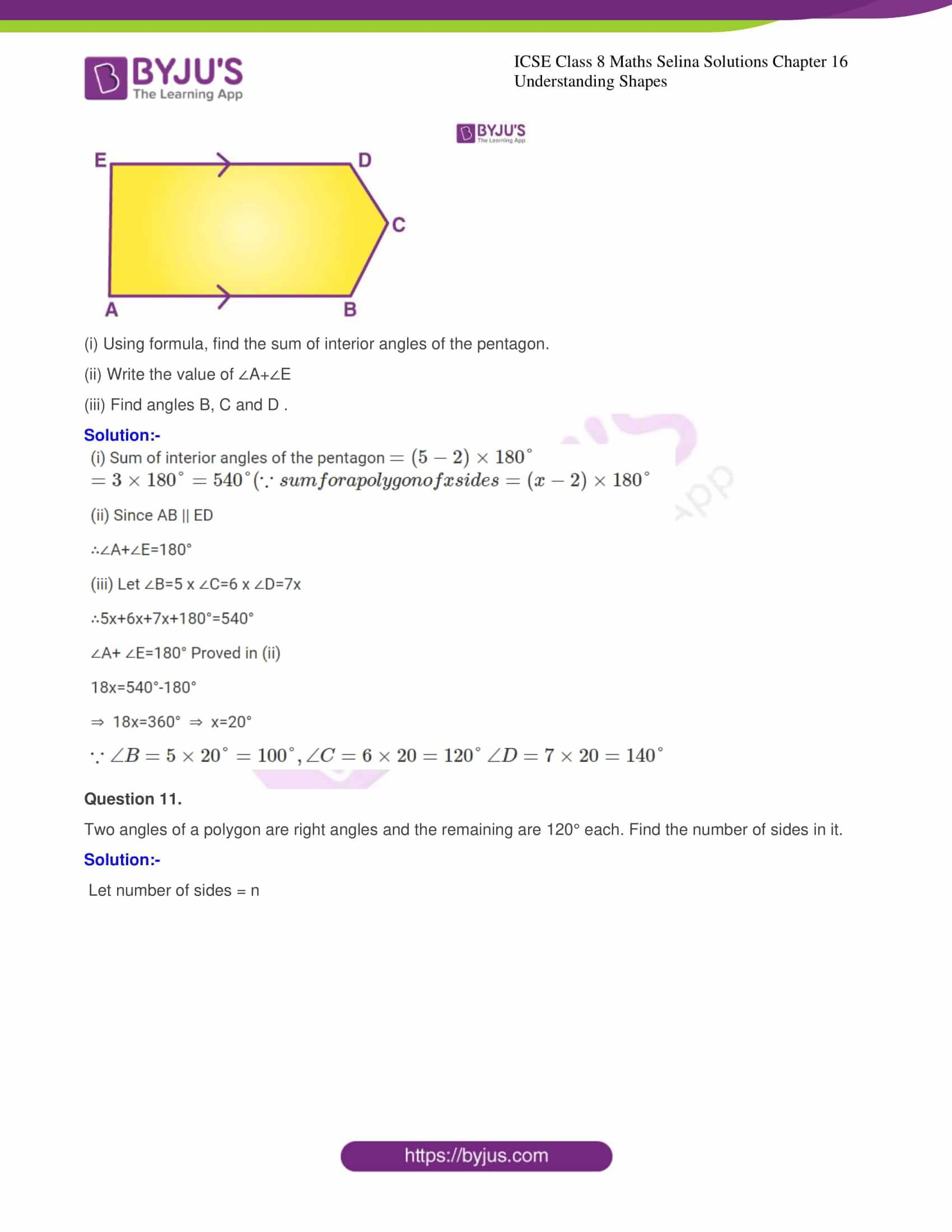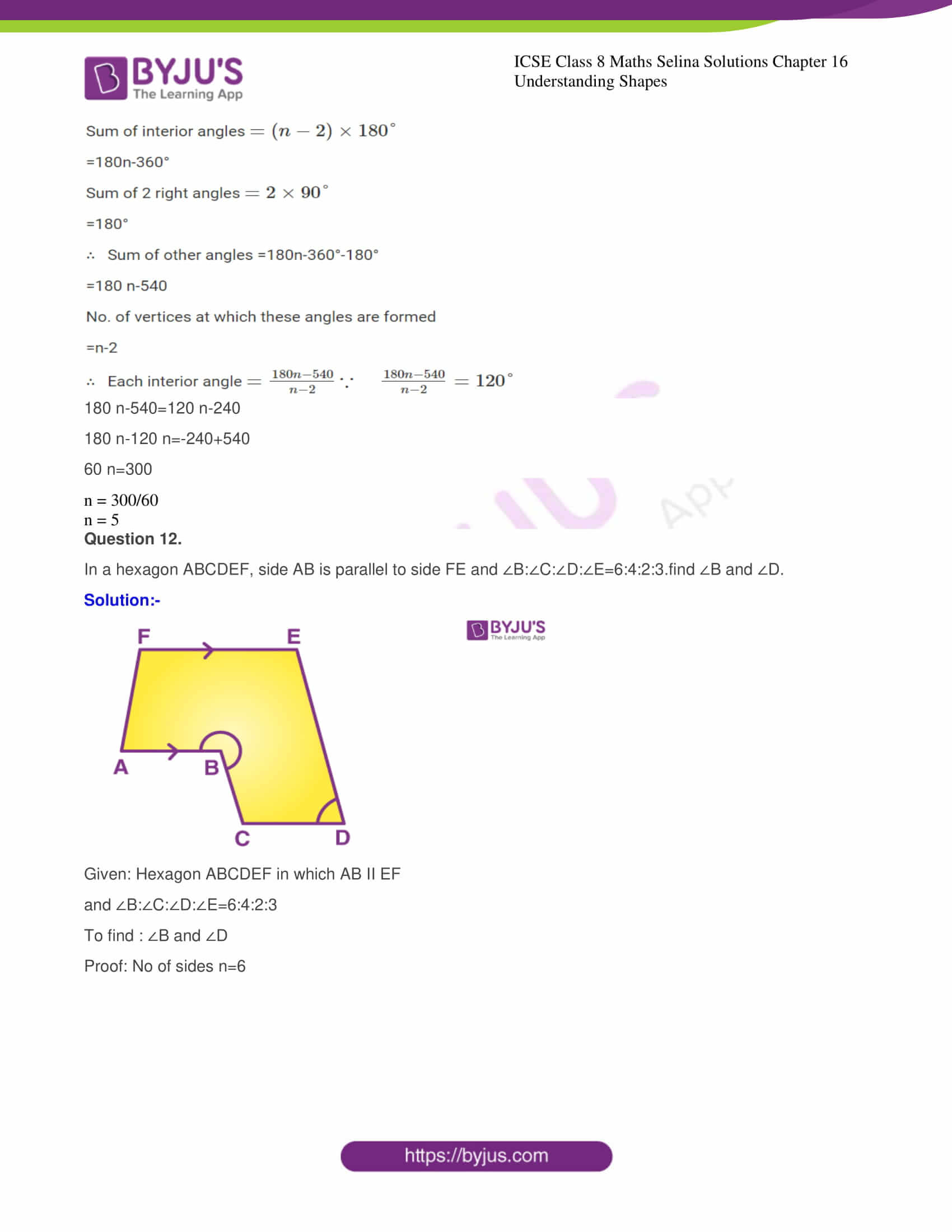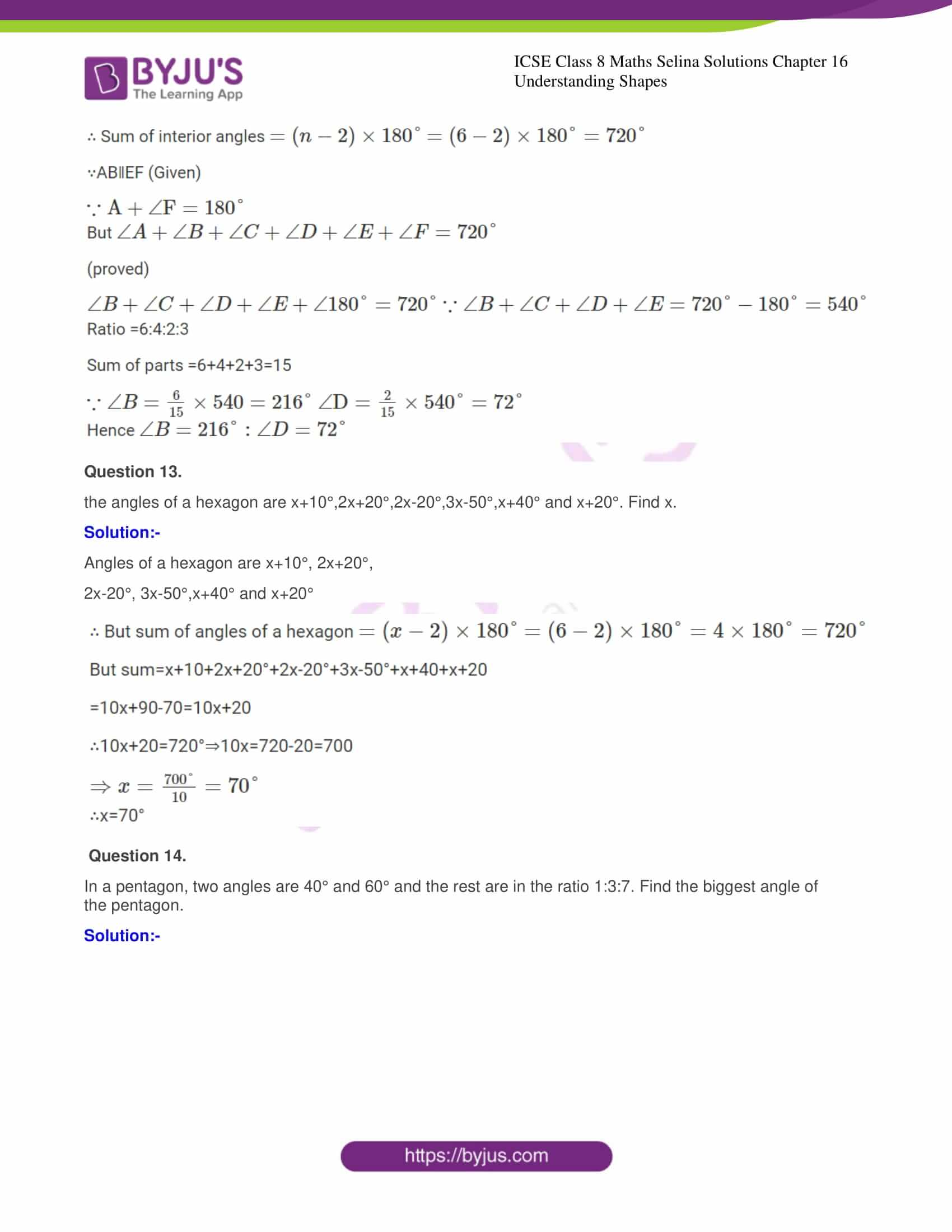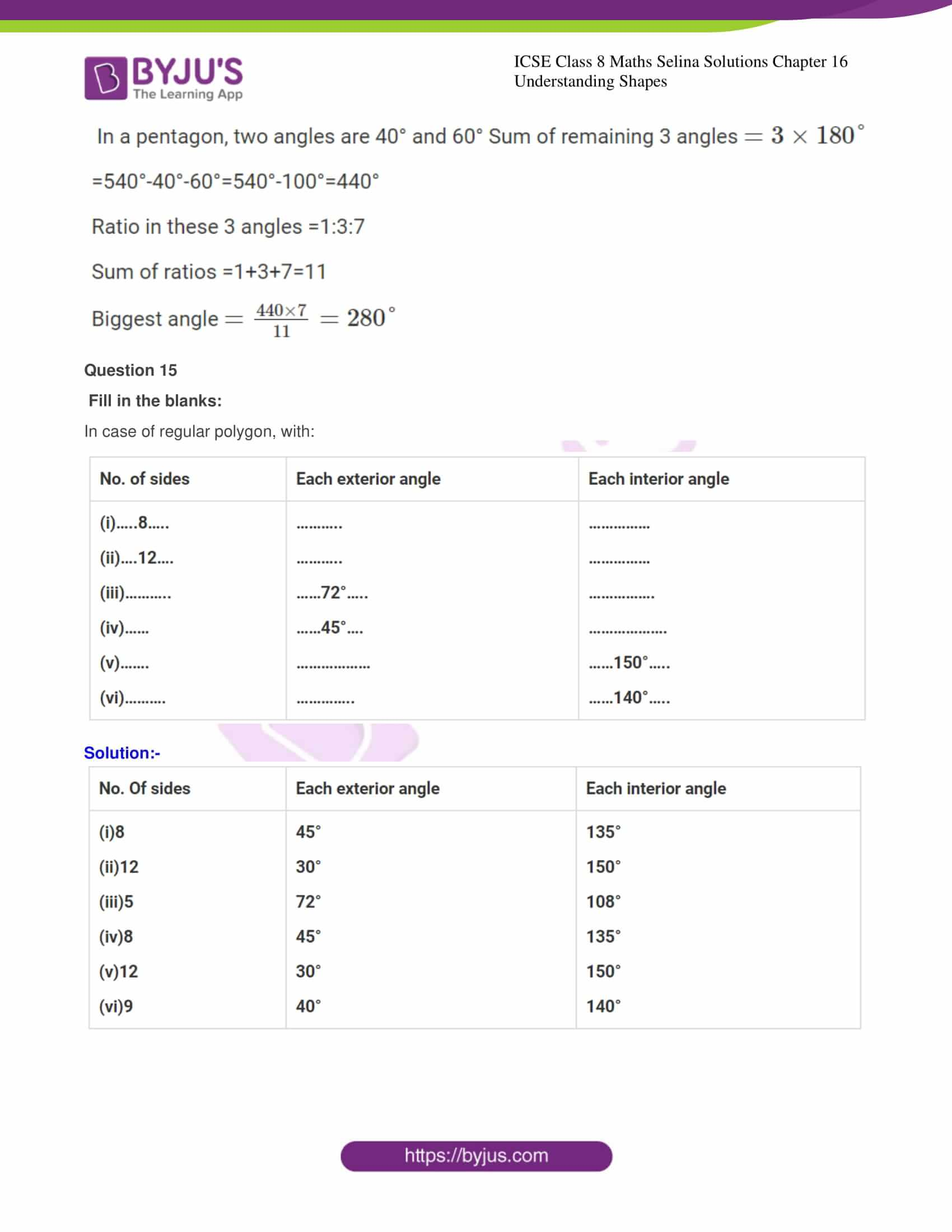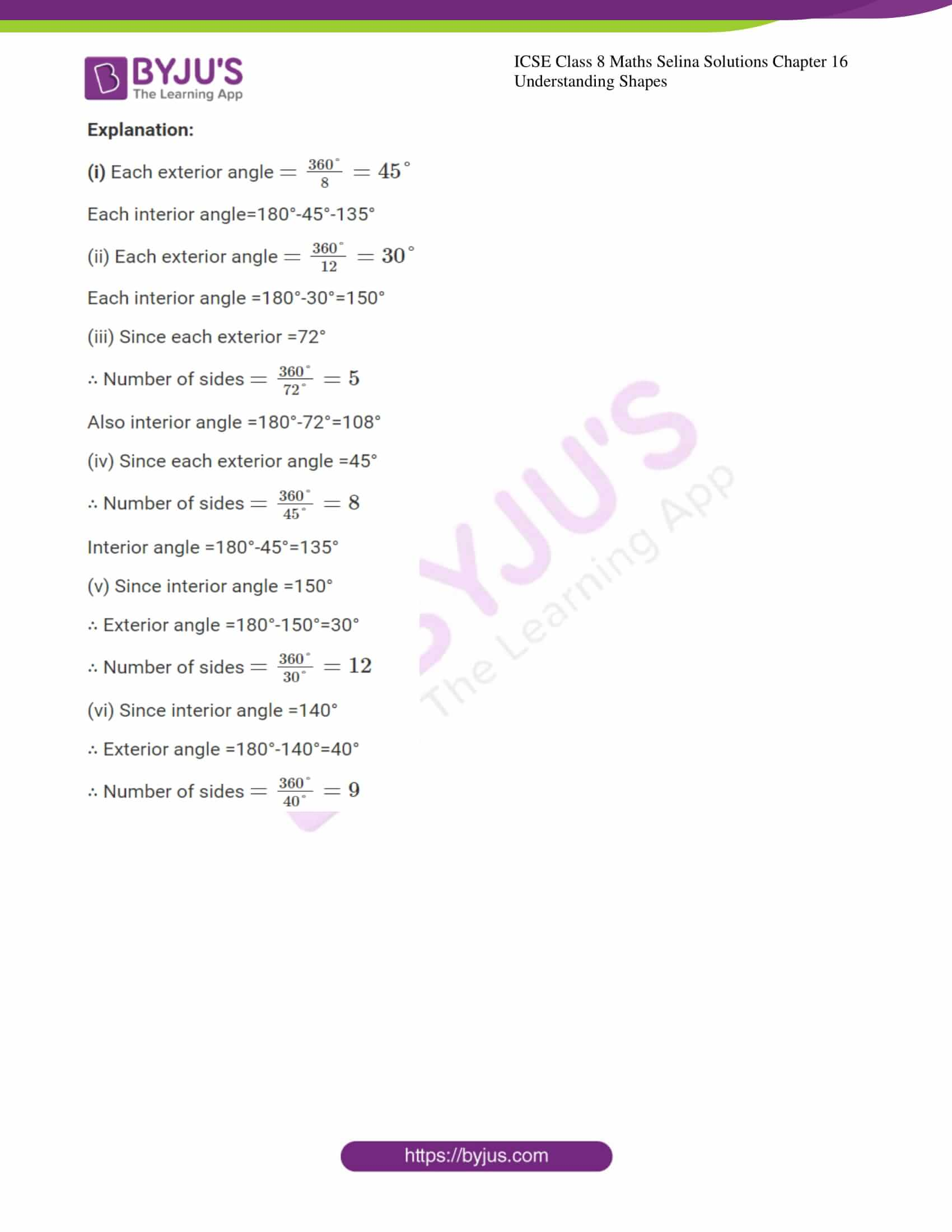Chapter 16 of ICSE Class 8 Maths Selina Solutions is based on the topic Understanding Shapes. This chapter consists of a total of 15 questions in one exercise. All the solutions are solved in a proper step by step method for easy understanding of the students.

### CHAPTER 16 – UNDERSTANDING SHAPES

Exercise

Question 1.

State which of the following are polygons: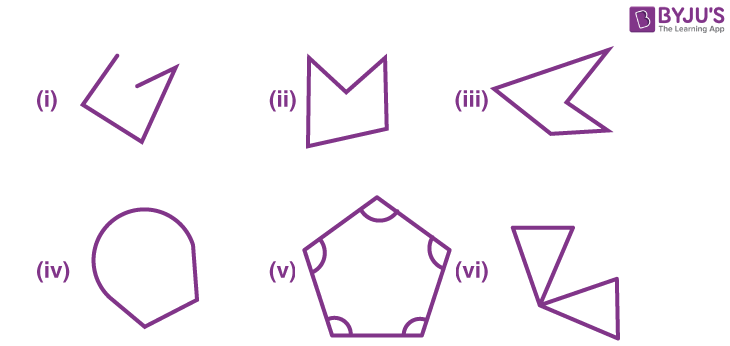If the given figure is a polygon, name it as convex or concave.

Solution:

In given Fig. (ii), (iii) and (v) are polygons.

Fig. (ii) and (iii) are concave polygons while

Fig. (v) is convex.

Question 2.

Calculate the sum of angles of a polygon with:

(i) 10 sides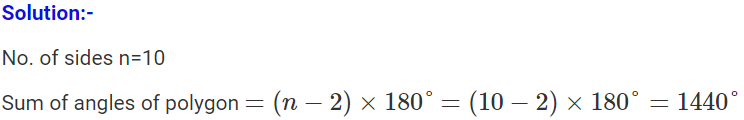(ii) 12 sides

Solution:-

No. of sides n=12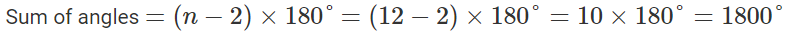(iii) 20 sides

Solution:-

n =20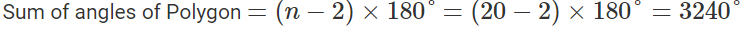(iv) 25 sides

Solution:-

n = 25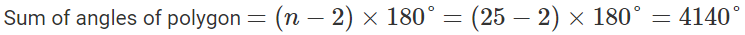Question 3.

Find the number of sides in a polygon if the sum of its interior angles is:

(i) 900°

Solution:-

Let no. of sides = n

Sum of angles of polygon= 900°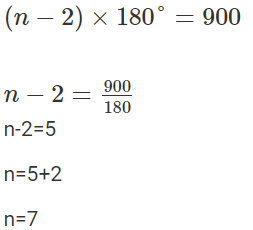(ii) 1620°

Solution:-

Let no. of sides = n

Sum of angles of polygon = 1620°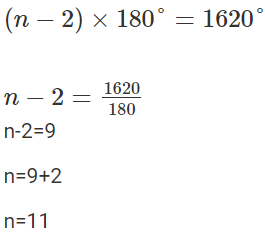(iii) 16 right-angles

Solution:-

Let no. of sides =n

Sum of angles of polygon =16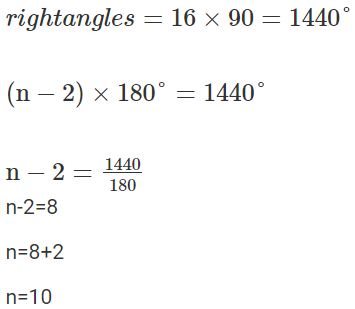(iv) 32 right-angles.

Solution:-

Let no. of sides =n

Sum of angles of polygon =32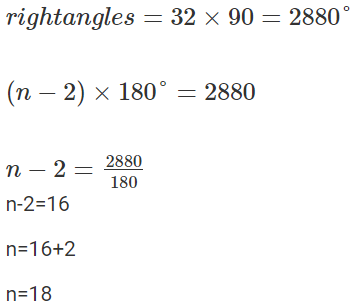Question 4.

Is it possible to have a polygon; whose sum of interior angles is?

(i) 870°

Solution:-

(i) Let no. of sides = n

Sum of angles =870°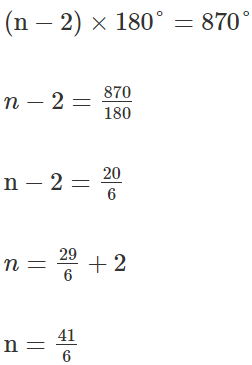Which is not a whole number.

Hence it is not possible to have a polygon, the sum of whose interior angles is 870°

(ii) 2340°

Solution:

Let no. of sides =n

Sum of angles =2340°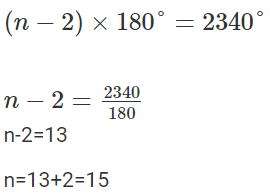Which is a whole number.

Hence it is possible to have a polygon, the sum of whose interior angles is 2340°.

(iii) 7 right-angles

Solution:-

Let no. of sides =n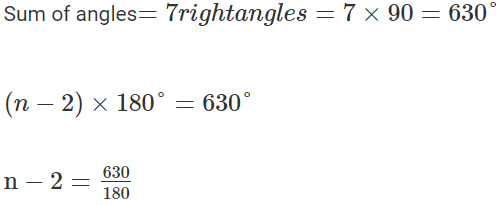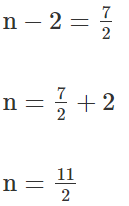Which is not a whole number. Hence it is not possible to have a polygon, the sum of whose interior angles is 7 right-angles.

(iv) 4500°

Solution:-

Let no. of sides = n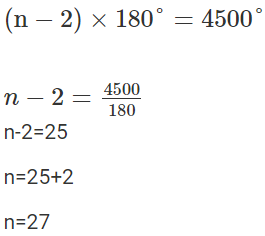Which is a whole number.

Hence it is possible to have a polygon, the sum of whose interior angles is 4500°.

Question 5.

(i) If all the angles of a hexagon are equal; find the measure of each angle.

Solution:-

No. of sides of hexagon, n=6

Let each angle be =x°

Sum of angles =6x°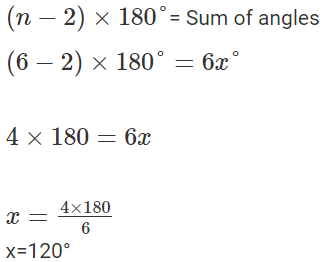∴ Each angle of hexagon =120°

(ii) If all the angles of a 14 – sided figure are equal; find the measure of each angle.

Solution:-

No. of sides of polygon, n=14

Let each angle =x°

Sum of angles =14x°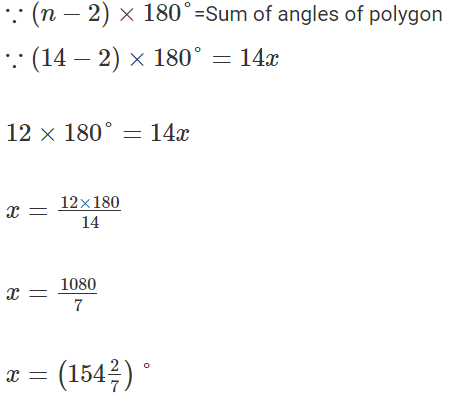Question 6.

Find the sum of exterior angles obtained on producing, in order, the sides of a polygon with:

(i) 7 sides

(ii) 10 sides

(iii) 250 sides.

(i) Solution:

No. of sides n=7

Sum of interior exterior angles at one vertex =180°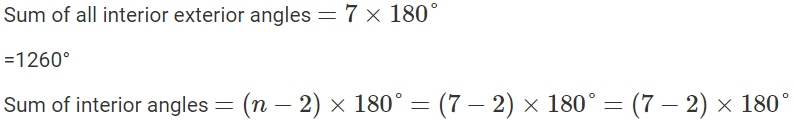=900°

∴Sum of exterior angles =1260°-900°

=360°

(ii)Solution

No. of sides n=10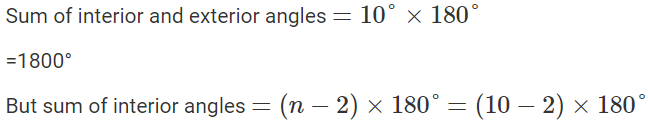=1440°

∴Sum of exterior angles  = 1800 – 1440

Sum of exterior angles = 360°

(iii)  Solution:

No. of side n=250

Sum of all interior and exterior angles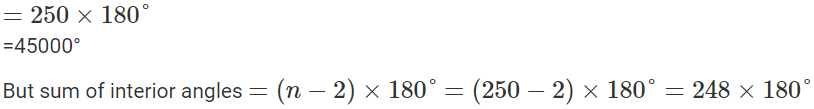=44640°

∴ Sum of exterior angles =45000-44640

=360°

Question 7 :

The sides of a hexagon are produced in order. If the measures of exterior angles so obtained are

(6x-1)° , (10 x+2)°,(8 x+2)°( 9 x-3)°,(5 x+4)° and  (12 x+6)°;Find each exterior angle.

Solution:-

Sum of exterior angles of hexagon formed by producing sides of order =360°

∴(6x-1)°+(10x+2)°+(8x+2)°+(9x-3)° +(5x+4)°+(12x+6)°=360°

50x+10°=360°

50x=360°-10°

50x=350°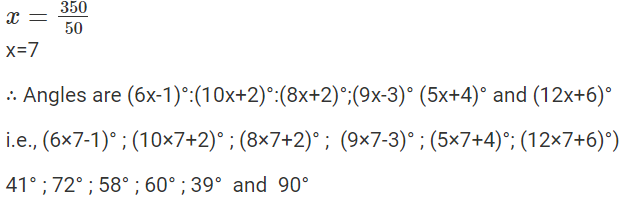Question 8.

The interior angles of a pentagon are in the ratio 4:5:6:7:5. Find each angle of the pentagon.

Solution:-

Let the interior angles of the pentagon be 4 x, 5 x, 6 x, 7 x, 5 x

Their sum = 4 x+5 x+6 x+7 x+5 x=27 x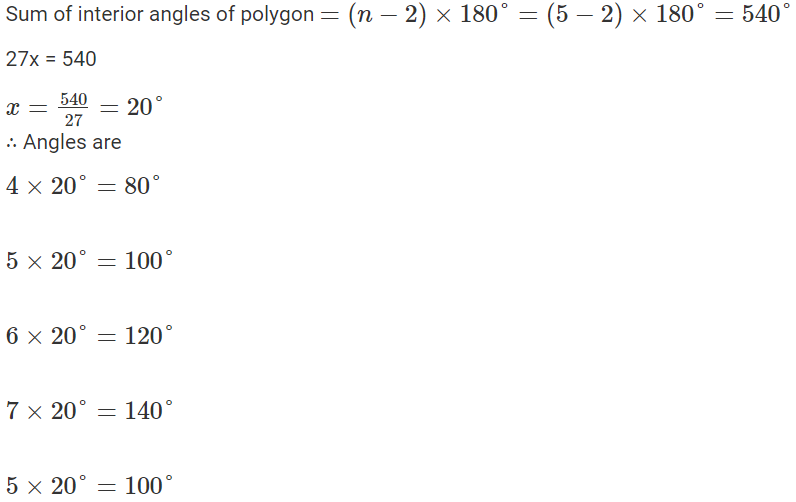Question 9

Two angles of a hexagon are 120°and 160°. If the remaining four angles are equal, find each equal angle.

Solution:-

Two angles of a hexagon are 120°, 160°

Let remaining four angles be x, x, x and x.

Their sum = 4 x+280°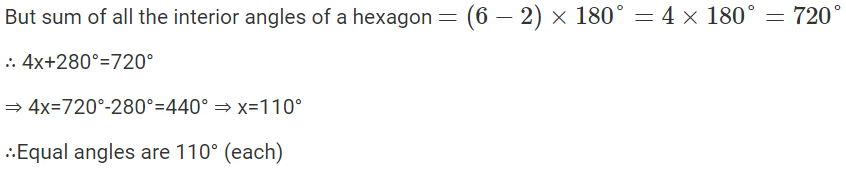Question 10

The figure, given below, shows a pentagon ABCDE with sides AB and ED parallel to each other, and

<B : <C : <D:5:6:7.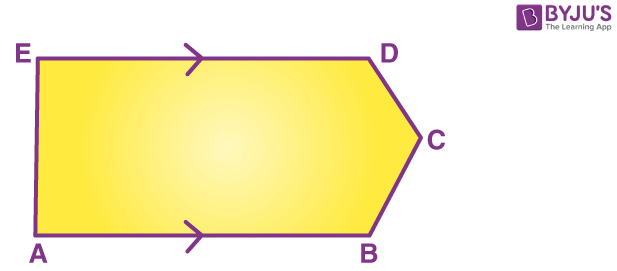(i) Using formula, find the sum of interior angles of the pentagon.

(ii) Write the value of ∠A+∠E

(iii) Find angles B, C and D .

Solution:-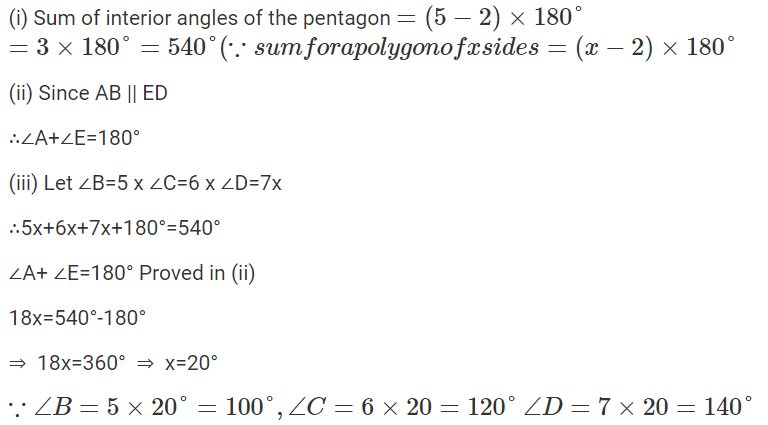Question 11.

Two angles of a polygon are right angles and the remaining are 120° each. Find the number of sides in it.

Solution:-

Let number of sides = n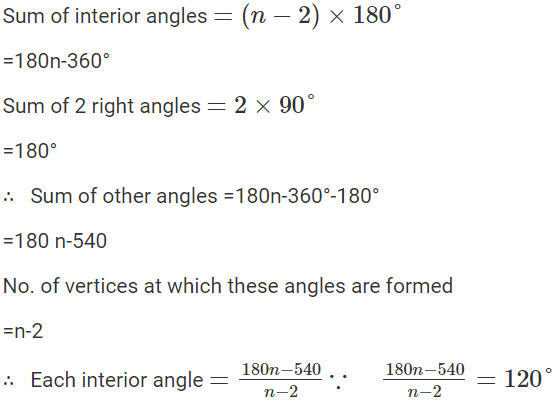180 n-540=120 n-240

180 n-120 n=-240+540

60 n=300

n = 300/60

n = 5

Question 12.

In a hexagon ABCDEF, side AB is parallel to side FE and ∠B:∠C:∠D:∠E=6:4:2:3.find ∠B and ∠D.

Solution:-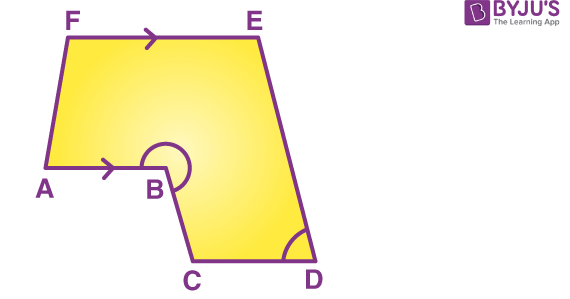Given: Hexagon ABCDEF in which AB II EF

and ∠B:∠C:∠D:∠E=6:4:2:3

To find : ∠B and ∠D

Proof: No of sides n=6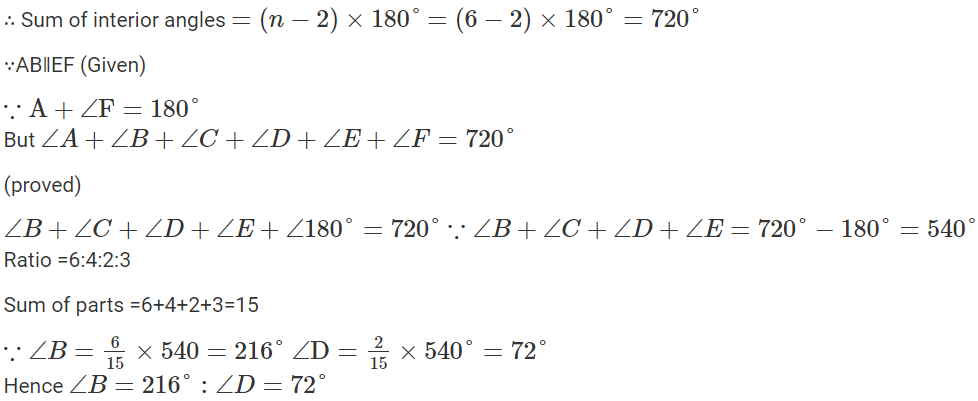Question 13.

the angles of a hexagon are x+10°,2x+20°,2x-20°,3x-50°,x+40° and x+20°. Find x.

Solution:-

Angles of a hexagon are x+10°, 2x+20°,

2x-20°, 3x-50°,x+40° and x+20°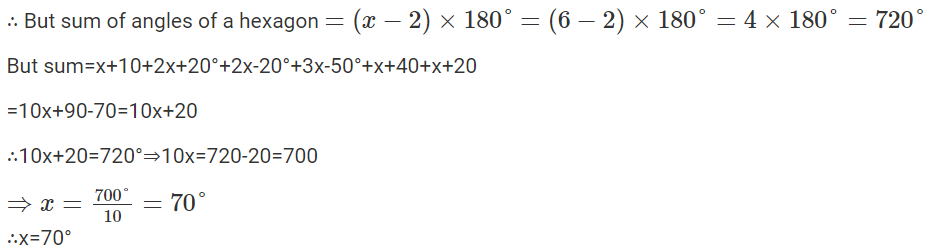Question 14.

In a pentagon, two angles are 40° and 60° and the rest are in the ratio 1:3:7. Find the biggest angle of the pentagon.

Solution:-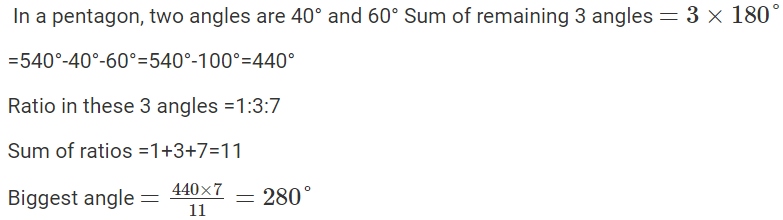Question 15

Fill in the blanks:

In case of regular polygon, with: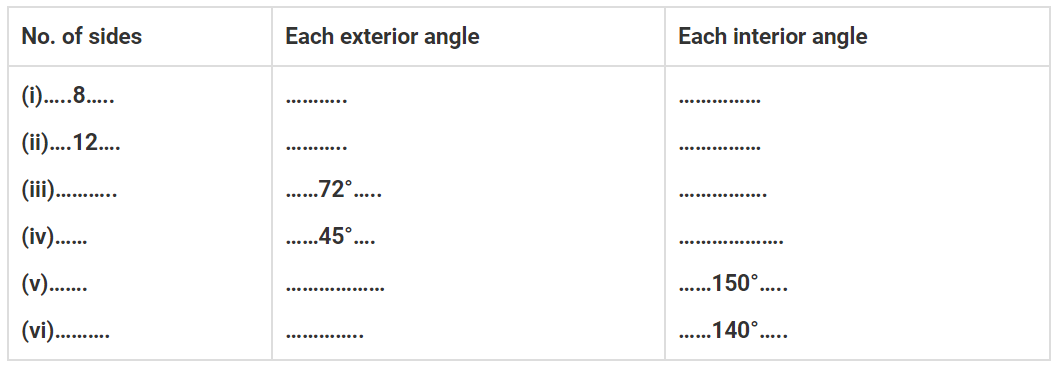Solution:-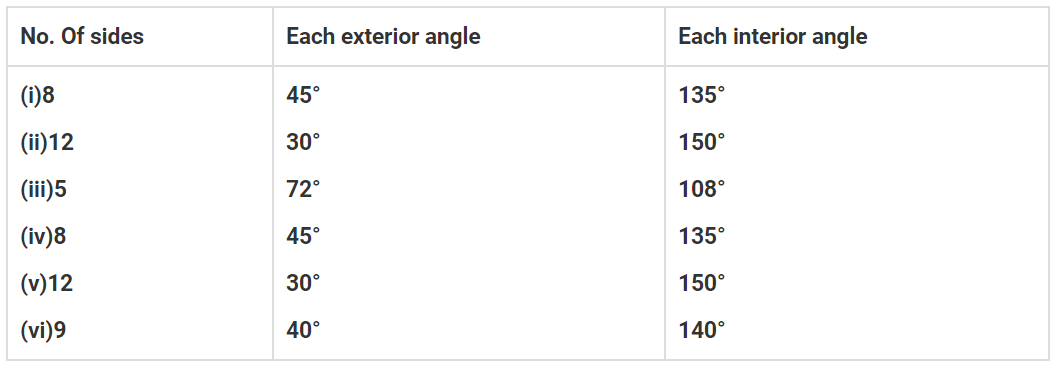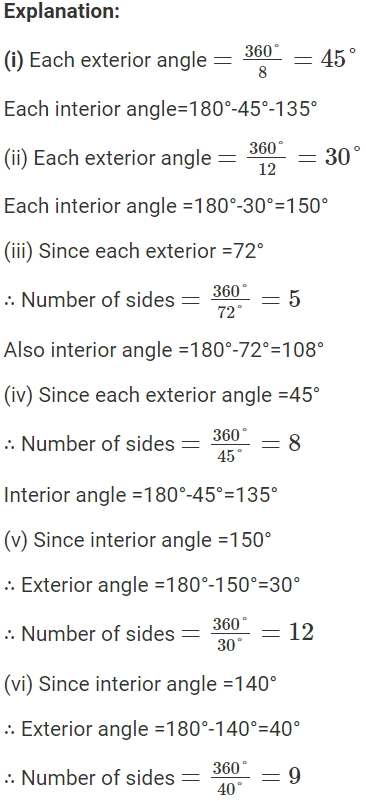### ICSE Class 8 Maths Selina Solutions Chapter 16 – Understanding Shapes

The concepts discussed in Chapter 16 – Understanding Shapes will help students to solve the problems related to the topic by using different types of theorems explained in the chapter. The topic comes under the unit of Geometry and it is important from exam point of view. This topic helps in understanding the world around us through different patterns, areas, volumes, lengths and angles.

For all the other subjects like Physics, Chemistry and Biology students can get the answers by clicking on ICSE Class 8 Selina Solutions.

We hope that the information provided here for ICSE Class 8 Maths Selina Solutions Chapter 16 Understanding Shapes will help students while preparing for their Class 8 final exam.